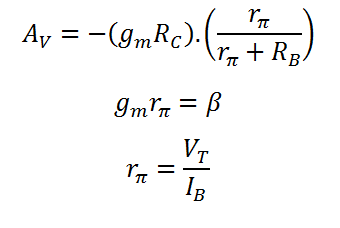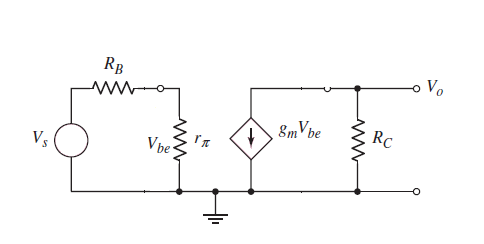# Common Emitter Amplifier Calculator (Small Signal Analysis)

This calculator computes the Diffusion Resistance (rp), Transconductance (gm) and Output Voltage gain (AV) for a common emitter (CE) BJT amplifier. In the common emitter mode the base terminal of the transistor is the input, the collector is the output and the emitter is common to both the base and collector. Common emitter amplifiers provide an inverted output with a high gain. The gain of this configuration is a function of temperature and the bias current.

*For self-biasing, VBB=VIN.(R2/R1+R2) and RB=(R1.R2)/(R1+R2)

** Enter T=300 K if nothing else is given.

K
mA
K-Ohm
K-Ohm

#### Result

K-Ohm
mA/V
V/V